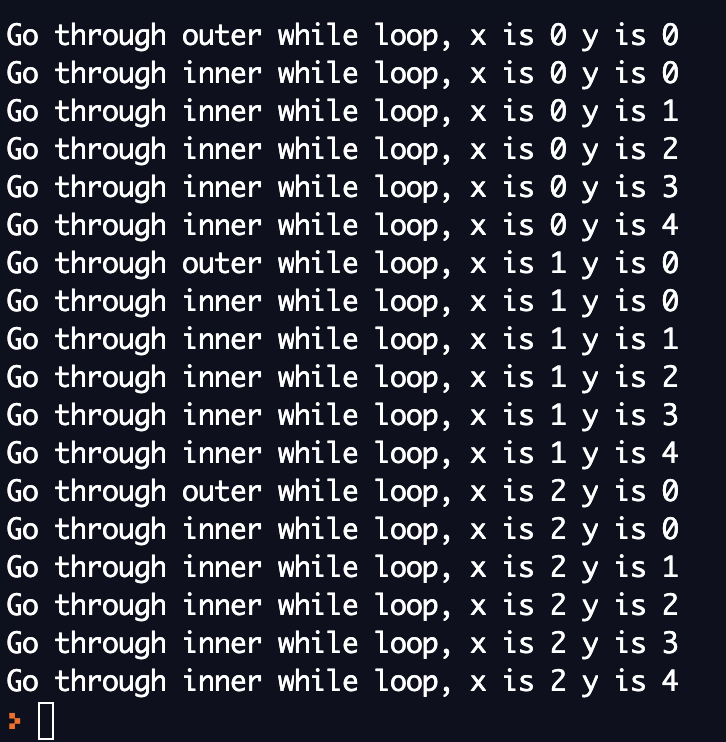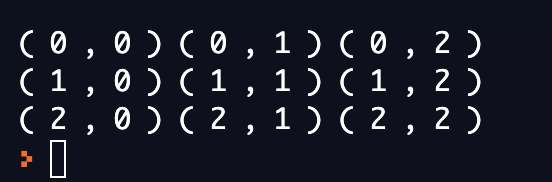# Nested loops

### Nested while loop

#This is the format for nested while loop
while expressionA:
while expressionB:
statement(B)
statement(A)


In the while loop above, when expressionA produces False, we will skip the whole block. When expressionA produces True, we will go to the inner while loop and check expressionB. If expressionB produces True, statement(B) will be executed. If expressionB produces False, we will skip the inner while loop and go to statement(A).
In conclusion, if expressionA produces False, none of the statements will be executed; statement(A) and statement(B) will both be executed if and only if both expressionA and expressionB produce True.

Let us take a look at one example for nested while loop.

#This is the example for nested while loop.
x = 0
y = 0
#Below is our outer loop
while x < 3:
#Print if "x < 3" produces True
print("Go through outer while loop, x is", x, "y is", y, sep = " ")
#Below is our inner loop
while y < 5:
#Print if "y < 5" produces True
print("Go through inner while loop, x is", x, "y is", y, sep = " ")
y += 1
#These codes below are executed after finishing each inner loop.
x += 1
y = 0


This is the output of our example.#### Questions

Can you try to find out how x and y values change and explain why?
(Hint: How many times do we went through the outer loop and inner loop separately?)

#### Challenge One

Try to change the integers in the nested while loop and predict the outputs. Then run the code and observe the outputs. Do they match your predictions?

### Nested for loop

#This is the format for nested for loop
for variableA in rangeA:
for variableB in rangeB:
statements(B)
statements(A)


In the for loop above, when variableA is not in rangeA, we will skip the whole block. When variableA is in rangeA, we will go to the inner for loop. If variableB is in rangeB, statement(B) will be executed. If variableB is not in rangeB, we will skip the inner for loop and and go to the outer loop.
In conclusion, if variableA is not in rangeA, none of the statements will be executed; statement(B) and statement(A) will both be executed if and only if variableA is in rangeA and variableB is in rangeB.

#This is the example for nested for loop
#Below is our outer loop
for n in range(0, 3):
#Below is our inner loop
for m in range(0, 3):
#Print if m is in range(0, 3)
print("(", n, ",", m, ")", end = " ")
#Print if n is in range(0, 3)
print("")


This is the output of our example.#### Questions

Can you try to explain the printing pattern?
(Hint: How many times do we went through the outer loop and inner loop separately?)

#### Challenge Two

Try to change the integers in the nested for loop and predict the outputs. Then run the code and observe the outputs. Do they match your predictions?

#### Questions

Try to initialize n and m. Will this change our output from the nested loops and why? Then run the code and observe the outputs. Do they match your predictions?

#### Questions

By the end of this section, let us think about the following questions: 1. Why initialization will not changing the output?
(Hint: What does the variable in the for loop represent?)
2. What should we do if we want start printing from (1, 1)?
(Hint: What would happen if we change the ranges?)